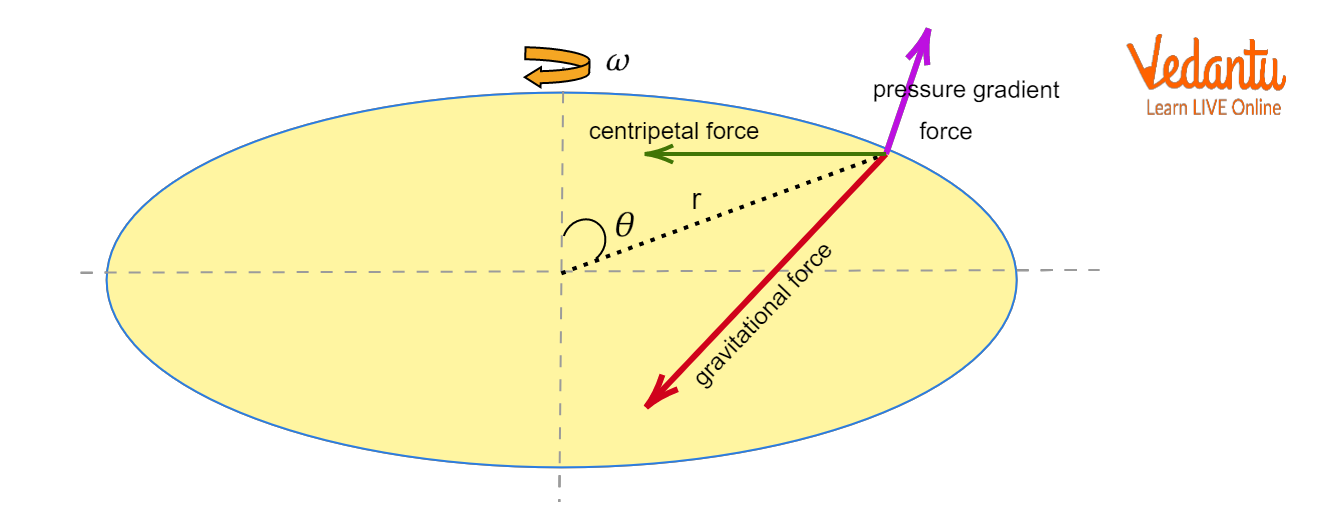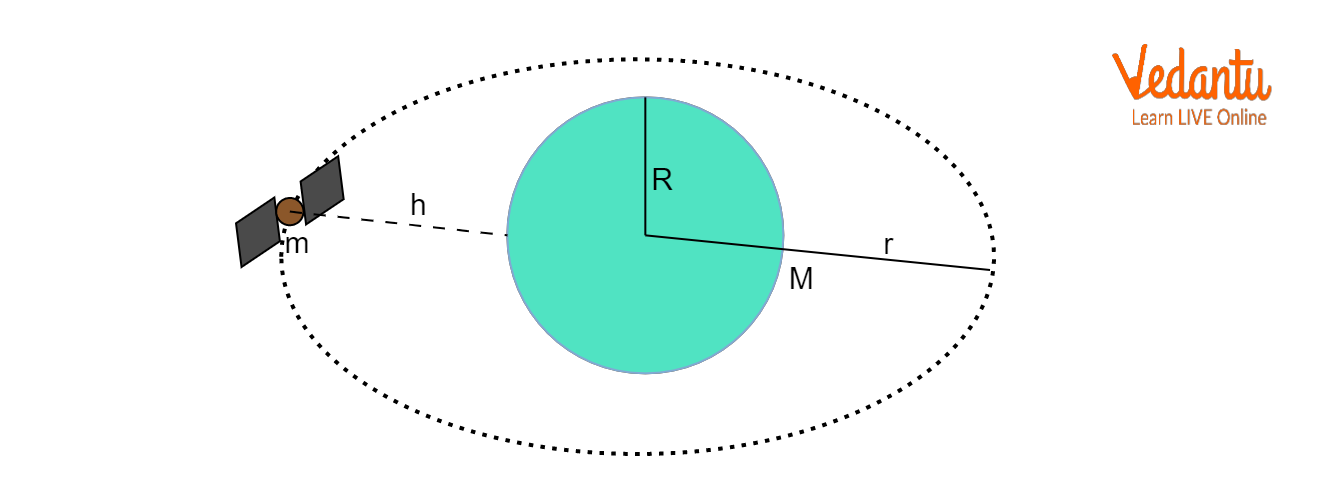Courses
Courses for Kids
Free study material
Free LIVE classes
More

# Orbital Velocity and Motion of Satellites - JEE Important TopicLIVE
Join Vedantu’s FREE Mastercalss

## What is Orbital Velocity?

Let us start by defining the orbital velocity of a satellite. The speed at which a body orbits another body is known as its orbital velocity. An orbit is a term used to describe an object's regular circular motion around the Earth. The distance between the object under consideration and the earth's centre determines the orbit's velocity. Due to their ability to orbit around any planet, artificial satellites are typically linked with this velocity. If the mass and radius of an object are known, the orbital velocity can be determined using the orbital velocity formula.

Due to its inertia, the moving body has a propensity to travel straight forward. On the other hand, the gravitational force tends to pull it downward. The elliptical or circular orbital path is a result of a balance between inertia and gravity. Orbital velocity is the speed at which a body must move in order to balance the forces of gravity and its own inertia. A satellite's altitude above the Earth affects the orbital velocity of the satellite as it travels around the planet. The closer it is to Earth, the quicker the necessary orbital velocity must be.

A satellite experiences drag when it runs across remnants of Earth's atmosphere at lower altitudes. The satellite gradually loses altitude due to this drag and falls back into the atmosphere where it burns up.

## How Satellites Work?

The velocity needed to maintain an orbit for a satellite, whether natural or artificial, is known as its orbital velocity. While gravitational force pulls the moving body down, inertia causes it to move in a straight line. Thus, the circular or elliptical orbital path demonstrates a balance between inertia and gravity.

A cannon shot fired from a mountaintop will travel farther if the muzzle velocity is increased. If the velocity is high enough, the bullet will never touch the ground. Imagine the projectile, or satellite, falling towards the Earth's surface while simultaneously imagining the Earth's surface curved away from it. The more massive the body in the centre of attraction is, the higher the orbital velocity for a given height or distance.

## Orbital Velocity Equation

The equation of the orbital velocity is given by:

${{V}_{orbit}}=\sqrt{\dfrac{GM}{r}}$

In the equation above, G stands for the gravitational constant, M for the body's central mass, and r for the orbit's radius.

If a planet's mass M and radius R are known, the orbital velocity equation can be used to determine its orbital velocity. Orbital Velocity is measured in metres per second (m/s). Orbital velocity ${{v}_{o}}$  is the speed at which the test mass orbits the source mass. The gravitational force, which is always an attracting force with its direction pointing towards the centre of a source mass, provides the centripetal force when the test mass is orbiting around the source mass in a circular path of radius "r" with the source mass's centre serving as the circular path's centre.Orbital Velocity

$\dfrac{mv_{o}^{2}}{r}=\dfrac{GMm}{{{r}^{2}}}$

$\Rightarrow \dfrac{v_{o}^{2}}{r}=\dfrac{GM}{{{r}^{2}}}$

$\Rightarrow {{v}_{o}}=\sqrt{\dfrac{GM}{r}}$

If the source mass and test mass are close together,

$r\approx R$ (radius of the source mass)

Then,

${{v}_{o}}=\sqrt{\dfrac{GM}{r}}$

According to the above formula, the orbital velocity is unrelated to the test mass. Orbital Velocity is measured in metres per second (m/s).

## Derive an Expression for Orbital Velocity Equation

We first need to understand the gravitational force and the centripetal force in order to calculate the orbital velocity. Understanding the gravitational pull is crucial since orbiting is made possible by it. To maintain the orbit of the orbiting body, a central body applies this gravitational pull to it. Due to its role in creating circular motion, centripetal force is very significant.

Let's use a satellite with mass m that orbits the Earth in a circle with a radius of r at a height of h from its surface as an example to illustrate how the formula is derived. If M and R are the Earth's mass and radius, respectively, then r=R+h.Satellite revolving around the Earth

To resolve the satellite, a centripetal force $\dfrac{mv_{o}^{2}}{r}$ is needed which is provided by the gravitational force of the $\dfrac{GMm}{{{r}^{2}}}$ acting between the satellite and the Earth.

Thus, on equating them, we get

$v_{o}^{2}=\dfrac{GM}{r}=\dfrac{GM}{R+h}$

Simplifying the above equation, we get

${{v}_{o}}=\sqrt{\dfrac{GM}{R+h}}$

Also, $GM=g{{R}^{2}}$ , where g is the acceleration due to gravity

${{v}_{o}}=\sqrt{\dfrac{g{{R}^{2}}}{R+h}}$

Simplifying the equation,

${{v}_{o}}=R\sqrt{\dfrac{g}{R+h}}$

Or, ${{v}_{o}}=\sqrt{\dfrac{GM}{r}}=\sqrt{\dfrac{GM}{R+h}}$........(1)

If g’ be the acceleration due to gravity at a height h from the surface.

Then  we have $g'=\dfrac{GM}{{{(R+h)}^{2}}}$

Now we can write, $g'(R+h)= \dfrac{GM}{R+h}$

Now substituting the value of $\dfrac{GM}{R+h}$ in terms of g’ in equation (1),

${{v}_{o}}=\sqrt{g'(R+h)}= \sqrt{g'\,r}$

When a satellite going around the earth in a circular orbit of radius r and speed v loses some of its energy, then

Thus we can observe that, when a satellite is going around the earth in a circular orbit any loss of energy will results in lower its radius and increase in velocity

## Orbital Velocity of Earth

The average speed of the Earth's orbit, in broad general terms, is 30 km/s, or 18$\dfrac{1}{2}$ mi/s.

## Geostationary Orbit Velocity

Geostationary orbit (GEO) satellites move at precisely the same speed as Earth to complete one full rotation of the planet above the equator in 23 hours 56 minutes and 4 seconds. This gives GEO satellites the appearance of being "stationary" over a specific location. The speed of GEO satellites should be around 3 km/s at an altitude of 35,786 km in order to properly match the rotation of the Earth. Compared to many satellites, this is far further away from the surface of the planet.

## Conclusion

The speed needed by a satellite to maintain equilibrium between its own inertia and the gravitational pull of the planet or celestial body it is orbiting is known as the orbital velocity. Orbital velocity is simply the speed or velocity needed for a satellite to continue orbiting a celestial body without losing speed. Additionally, the satellite always orbits a celestial body with a mass bigger than its own. The more massive the body in the centre of attraction is, the higher the orbital velocity for a given height or distance.

Last updated date: 17th Sep 2023
Total views: 123.9k
Views today: 2.23k

## FAQs on Orbital Velocity and Motion of Satellites - JEE Important Topic

1. What factors affect orbital velocity?

The curved surface of a planet, star, or other celestial body allows for orbital velocity. An orbiting item travels in a straight line, but the body it orbits curves. The constant curvature of the orbited body prevents the orbiting object from falling all the way to the surface, provided it keeps the proper speed. It is simpler to maintain a constant speed in space than it is on Earth because of the concept of inertia.

An item in motion tends to continue in motion unless acted on by an outside force, according to one of Sir Isaac Newton's laws of inertia. The speed of a flying object is slowed as it travels through the sky because of collisions with numerous air molecules in the earth's atmosphere. As you ascend above the earth's atmosphere, the air becomes more empty and has less molecules to hinder a circling spacecraft's forward motion.

2. How do satellites work?

The velocity needed to maintain an orbit for a satellite, whether natural or artificial, is known as its orbital velocity. While gravitational force pulls the moving body down, inertia causes it to move in a straight line. Thus, the circular or elliptical orbital path demonstrates a balance between inertia and gravity. A cannon shot fired from a mountaintop will travel farther if the muzzle velocity is increased. If the velocity is high enough, the bullet will never touch the ground. Imagine the projectile, or satellite, falling towards the Earth's surface while simultaneously imagining the Earth's surface curved away from it. The more massive the body in the centre of attraction is, the higher the orbital velocity for a given height or distance.